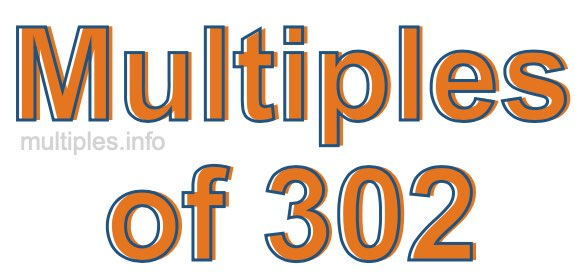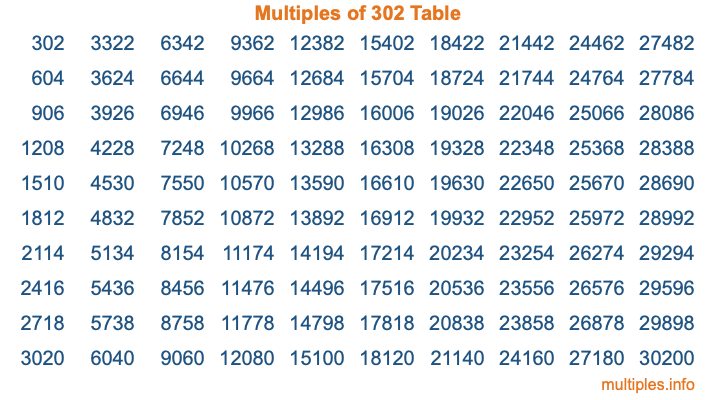Multiples of 302Welcome to the Multiples of 302 page. Here we will first teach you everything you will ever need to know about the multiples of 302, and then give you a study guide summary of everything we taught you to make sure you remember it all. Use this page to look up facts and learn information about the multiples of 302. This page will make you a multiples of three hundred two expert!

Definition of Multiples of 302
Multiples of 302 are all the numbers that when divided by 302 equal an integer. Each of the multiples of 302 are called a multiple. A multiple of 302 is created by multiplying 302 by an integer.

Therefore, to create a list of multiples of 302, you start with 1 multiplied by 302, then 2 multiplied by 302, then 3 multiplied by 302, and so on for as long as you want. Thus, the list of the first five multiples of 302 is 302, 604, 906, 1208, and 1510. To see a larger list of multiples of 302, see the printable image of Multiples of 302 further down on this page. We also have a category where you can choose any nth multiple of 302.

Multiples of 302 Checker
The Multiples of 302 Checker below checks to see if any number of your choice is a multiple of 302. In other words, it checks to see if there is any number (integer) that when multiplied by 302 will equal your number. To do that, we divide your number by 302. If the the quotient is an integer, then your number is a multiple of 302.

Is  a multiple of 302?

Least Common Multiple of 302 and ...
A Least Common Multiple (LCM) is the lowest multiple that two or more numbers have in common. This is also called the smallest common multiple or lowest common multiple and is useful to know when you are adding our subtracting fractions. Enter one or more numbers below (302 is already entered) to find the LCM.

Check out our LCM Calculator if you need more details about the Least Common Multiple or if you need the LCM for different numbers for adding and subtraction fractions.

nth Multiple of 302
As we stated above, 302 is the first multiple of 302, 604 is the second multiple of 302, 906 is the third multiple of 302, and so on. Enter a number below to find the nth multiple of 302.

th multiple of 302

Multiples of 302 vs Factors of 302
302 is a multiple of 302 and a factor of 302, but that is where the similarities end. All postive multiples of 302 are 302 or greater than 302. All positive factors of 302 are 302 or less than 302.

Below is the beginning list of multiples of 302 and the factors of 302 so you can compare:

Multiples of 302: 302, 604, 906, 1208, 1510, etc.

Factors of 302: 1, 2, 151, 302

As you can see, the multiples of 302 are all the numbers that you can divide by 302 to get a whole number. The factors of 302, on the other hand, are all the whole numbers that you can multiply by another whole number to get 302.

It's also interesting to note that if a number (x) is a factor of 302, then 302 will also be a multiple of that number (x).

Multiples of 302 vs Divisors of 302
The divisors of 302 are all the integers that 302 can be divided by evenly. Below is a list of the divisors of 302.

Divisors of 302: 1, 2, 151, 302

The interesting thing to note here is that if you take any multiple of 302 and divide it by a divisor of 302, you will see that the quotient is an integer.

Multiples of 302 Table
Below is an image of the first 100 multiples of 302 in a table. The table is in chronological order, column by column. The first column has the first ten multiples of 302, the second column has the next ten multiples of 302, and so on.The Multiples of 302 Table is also referred to as the 302 Times Table or Times Table of 302. You are welcome to print out our table for your studies.

Negative Multiples of 302
Although not often discussed or needed in math, it is worth mentioning that you can make a list of negative multiples of 302 by multiplying 302 by -1, then by -2, then by -3, and so on, to get the following list of negative multiples of 302:

-302, -604, -906, -1208, -1510, etc.

Multiples of 302 Summary
Below is a summary of important Multiples of 302 facts that we have discussed on this page. To retain the knowledge on this page, we recommend that you read through the summary and explain to yourself or a study partner why they hold true.

There are an infinite number of multiples of 302.

A multiple of 302 divided by 302 will equal a whole number.

302 divided by a factor of 302 equals a divisor of 302.

The nth multiple of 302 is n times 302.

The largest factor of 302 is equal to the first positive multiple of 302.

302 is a multiple of every factor of 302.

302 is a multiple of 302.

A multiple of 302 divided by a divisor of 302 equals an integer.

302 divided by a divisor of 302 equals a factor of 302.

Any integer times 302 will equal a multiple of 302.

Multiples of a Number
Here you can get the multiples of another number, all with the same attention to detail as we did for multiples of 302 on this page.

Multiples of
Multiples of 303
Did you find our page about multiples of three hundred two educational? Do you want more knowledge? Check out the multiples of the next number on our list!